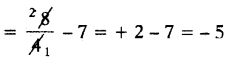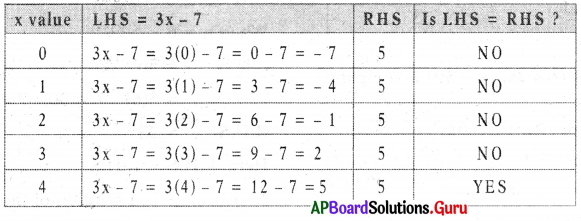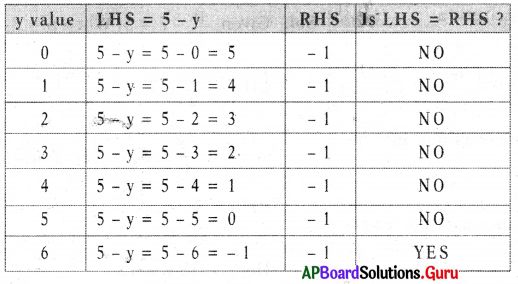# AP Board 7th Class Maths Solutions Chapter 3 Simple Equations Ex 3.1

SCERT AP 7th Class Maths Solutions Pdf Chapter 3 Simple Equations Ex 3.1 Textbook Exercise Questions and Answers.

## AP State Syllabus 7th Class Maths Solutions 3rd Lesson Simple Equations Exercise 3.1

Question 1.
Write the equations of the following mathematical statements.
(i) A number x decreased by 5 is 14.
Given number = x
Number decreased by 5 = x – 5
∴ x – 15 = 14.(ii) Eight times of y plus 3 is -5.
Given number = y
Eight times of y = 8 ∙ y
Eight times of y plus 3 = 8y + 3
∴ 8y + 3 = – 5

(iii) If you add one fourth of z to 3 you get 7.
Given number = z
One fourth of z = $$\frac{1}{4}$$ ∙ z = $$\frac{z}{4}$$
One fourth of z is added to 3 = $$\frac{z}{4}$$ + 3
Result is 7
∴ $$\frac{z}{4}$$ + 3 = 7.

(iv) If you take away 5 from 3 times of m, you get 11.
Given number = m
3 times of m = 3m
Take away 5 from 3 times of m = 3m – 5
Result is 11
∴ 3m – 5 = 11

(v) Sum of angles 2x, (x – 30) is a right angle.
Given angles 2x, (x – 30)
Sum of angles 2x, (x – 30)
= 2x + x – 30 = 3x – 30
Sum of angles is right angle (90°).
∴ 3x – 30 = 90°

(vi) The perimeter of a square of side ‘a’ is 14 m.
Given side of a square = a
Perimeter = 4 ∙ side = 4 ∙ a
Given perimeter = 14 m
∴ 4a = 14 mQuestion 2.
Write the following equations in statement form.
(i) m – 5 = 12
A number m is decreased by 5 is 12.

(ii) $$\frac{\mathbf{a}}{\mathbf{3}}$$ = 4
One third of a is 4.

(iii) 4x + 7 = 15
Sum of 4 times of x and 7 is 15.
(or)
7 is added to 4 times of x is 15.

(iv) 2 – 3y = 11
2 is decreased by 3 times of y is 11.
(or)
3 times of y is subtracted from 2 is 11.

Question 3.
Check whether the value given in the brackets is a solution to the given equation or not.
(i) 5n – 7 = 23 (n = 6)
Given 5n – 7 = 23
When n = 6
L.H.S = 5n – 7
= 5(6) – 7
= 30 – 7
= 23
R.H.S =23
Here, L.H.S = R.H.S
So, n = 6 is a solution of the given equation.(ii) $$\frac{p}{4}$$ – 7 = 5 (p = 8)
Given $$\frac{p}{4}$$ – 7 = 5; When p = 8
LHS = $$\frac{p}{4}$$ – 7RHS = 5
– 5 ≠ 5
Here, LHS ≠ RHS
So, p = 8 is not a solution of the given equation.

(iii) 5 – 2x = 19 -7
Given 5 – 2x = 19
When x = – 7
LHS = 5 – 2x
= 5 – 2(- 7) = 5 + 14 = 19
RHS = 19
19 = 19
Here, LHS = RHS
So, x = – 7 is a solution of the given equation.

(iv) 2 + 3(m – 1) = 5 (m = -2)
Given 2 + 3(m – 1) = 5
When m = – 2
LHS = 2 + 3(m – 1)
= 2 + 3(- 2 – 1)
= 2 + 3(- 3) = 2 – 9 = – 7
RHS = 5
– 7 ≠ 5
Here, LHS ≠ RHS
So, m = – 2 is not a solution of given equation.Question 4.
Solve the following equations using trial and error method,
(i) 3x – 7 = 5